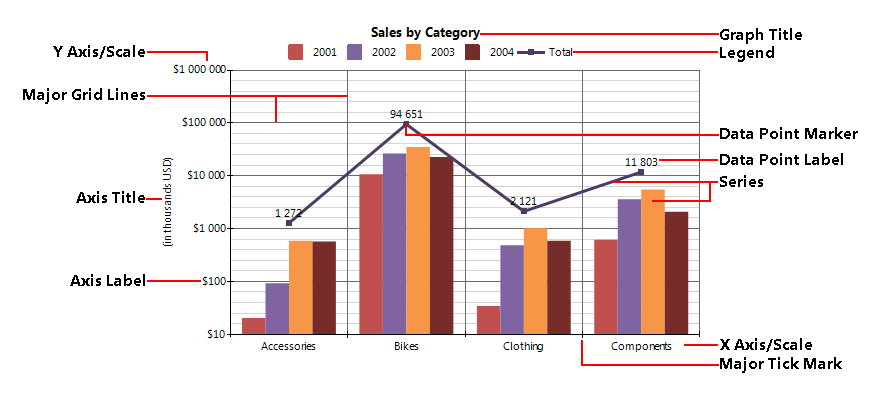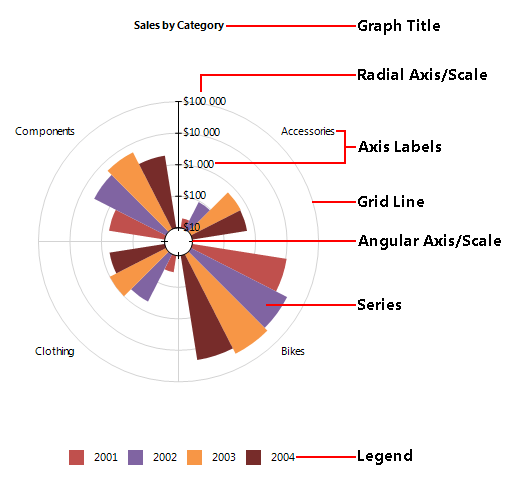In the previous post Impress users with new visualizations in Telerik Reporting Q1 2013, we covered some of the features that the new Graph report item offers to developers who want to add data visualizations to Telerik reports. Just to recap, the new Graph report item uses the crosstab’s OLAP data engine, and offers a set of new visualization capabilities which will help your users understand data quicker and make better decisions.

But what is really different in the new Graph item and how it is to be used? Let’s dig into its integral parts and powerful features, which will allow you to create amazing OLAP (and not only) charts quicker than ever before. Most of this blog would be chart theory and may sound familiar, but we will go over it anyway :)

Fig. 1. Combined Bar and Line charts in a Graph item with Cartesian coordinate system:Fig. 2. Rose (Bar) chart in a Graph item with Polar coordinate systemCoordinate systems
The Graph item uses a two-dimensional coordinate system that uniquely identifies the position of each data point (http://en.wikipedia.org/wiki/Coordinate_system). Each coordinate system consists of two reference lines called coordinate axes or just axes and an origin

The Graph item supports two types of two-dimensional coordinate systems:

The data point in the Cartesian coordinate system is represented by an ordered pair of two coordinates (x, y).

• Polar (http://en.wikipedia.org/wiki/Polar_coordinate_system) is used where each point on a plane is determined by a distance from the origin (called the radial coordinate or radius) and an angle from a fixed direction (the angular coordinate, polar angle, or azimuth). The polar coordinate system has two axes:
• angular axis - the circular axis for the angular coordinate;

The data point in the Polar coordinate system is represented by an ordered pair of two coordinates (r, ϴ).

Since there is a direct conversion between the two coordinate systems they are interchangeable in the Graph item. The coordinates of the data points in the Graph are represented by the (x, y) pair that for the Polar coordinate system is converted to (ϴ, r):

(x, y) ⇔ (ϴ, r).

The coordinate system also defines the default appearance/style of the two axes.

The Axis
The coordinate axis or simply axis represent a single dimension of the coordinate system.
An axis consists of:

• Scale - defines how the data is projected on the axis;
• Tick marks - major/minor - periodic graduations;
• Labels - numerical/categorical indications accompanying the tick marks;
• Title - the title of the axis, usually a brief description of the dimension;
• Grid lines - Within the graph a grid of lines may appear to aid in the visual alignment of data. The grid can be enhanced by visually emphasizing the lines at regular or significant graduations. The emphasized lines are then called major grid lines and the remainder are minor grid lines.

Scales
define how the data is projected on the corresponding axis that is how the data from the user domain is converted to coordinates.
According to the type of the input data there are 5 types of scales:

• NumericalScale - represents a scale with continuous domain of numbers: integer numbers (Int16, Int32, Int64) or floating point numbers (Single, Double), etc;
• LogarithmicScale - a numerical scale that applies a logarithmic transformation (with a given base) to the input data;
• ExponentialScale - a numerical scale that applies an exponential transformation (with a given power) to the input data;
• DateTimeScale - represents a scale with continuous domain of DateTime values;
• CategoryScale - represents an ordinal scale with discrete domain like names and categories.

Series
- a series of data points that represents individual measurements. The graph item supports:

• Bar (clustered, stacked, stacked 100%). Used for Bar, Column, Pie and Rose chart.
• Line (stacked or stacked 100%). Used for for Line, Bubble, Radar line and Spider line charts.
• Area (stacked or stacked 100%). Used for Area, Radar area and Spider area charts.
• Range Bar
• Range Area

Legend
When the data appearing in a chart contains multiple variables, the chart may include a legend. The legend contains a list of the variables appearing in the chart and an example of their appearance. This information allows the data from each variable to be identified in the graph.

Title
The Graph item can have one or more Titles that provides a succinct description of what the data in the graph refers to.

Stay tuned
This is the first blog of a series where we will introduce the new Telerik Reporting Graph item powerful features.